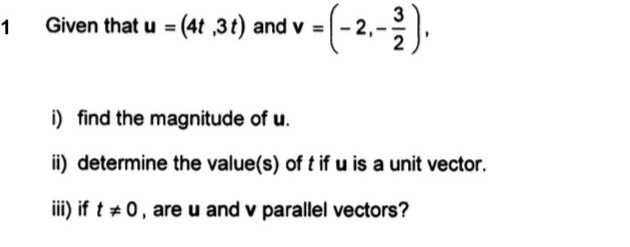### ¿Todavía tienes preguntas de matemáticas?

Pregunte a nuestros tutores expertos
Algebra
Pregunta$$1$$ Given that $$u = ( 4 t , 3 t )$$ and $$v = ( - 2 , - \frac { 3 } { 2 } )$$

i) find the magnitude of $$u$$ . ii) determine the value(s) of $$t$$ if $$u$$ is a unit vector. iii) if $$t \neq 0$$ , are $$u$$ and $$v$$ parallel vectors?

i) $$\sqrt { ( 4 t ) ^ { 2 } + B t ) ^ { 2 } } = 5 t$$
ii) $$5 t = 1 t = \frac { 1 } { 5 }$$
(iii) $$\left. \begin{array} { l }{ k _ { u } = \frac { 3 t } { 4 t } = \frac { 3 } { 4 } }\\{ k _ { v } = \frac { - \frac { 3 } { 2 } } { - 2 } = \frac { 3 } { 2 } \times \frac { 1 } { 2 } = \frac { 3 } { 4 } } \end{array} \right.$$
$$k _ { u } = k _ { v }$$
$$\therefore$$ They are parallel vectors.